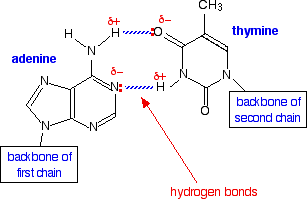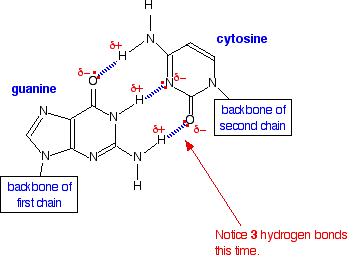# 11.5: Hydrogen Bonds

$$\newcommand{\vecs}{\overset { \rightharpoonup} {\mathbf{#1}} }$$ $$\newcommand{\vecd}{\overset{-\!-\!\rightharpoonup}{\vphantom{a}\smash {#1}}}$$$$\newcommand{\id}{\mathrm{id}}$$ $$\newcommand{\Span}{\mathrm{span}}$$ $$\newcommand{\kernel}{\mathrm{null}\,}$$ $$\newcommand{\range}{\mathrm{range}\,}$$ $$\newcommand{\RealPart}{\mathrm{Re}}$$ $$\newcommand{\ImaginaryPart}{\mathrm{Im}}$$ $$\newcommand{\Argument}{\mathrm{Arg}}$$ $$\newcommand{\norm}{\| #1 \|}$$ $$\newcommand{\inner}{\langle #1, #2 \rangle}$$ $$\newcommand{\Span}{\mathrm{span}}$$ $$\newcommand{\id}{\mathrm{id}}$$ $$\newcommand{\Span}{\mathrm{span}}$$ $$\newcommand{\kernel}{\mathrm{null}\,}$$ $$\newcommand{\range}{\mathrm{range}\,}$$ $$\newcommand{\RealPart}{\mathrm{Re}}$$ $$\newcommand{\ImaginaryPart}{\mathrm{Im}}$$ $$\newcommand{\Argument}{\mathrm{Arg}}$$ $$\newcommand{\norm}{\| #1 \|}$$ $$\newcommand{\inner}{\langle #1, #2 \rangle}$$ $$\newcommand{\Span}{\mathrm{span}}$$$$\newcommand{\AA}{\unicode[.8,0]{x212B}}$$

## Introduction

Figure $$\PageIndex{1}$$: In this rotating model oxygen are red, carbon grey and hydrogen white.

Hydrogen bonds are a strong type of dipole-dipole interaction. As a Rule of Thumb, they are weaker than covalent and ionic ("intramolecular") bonds", but stronger than most dipole-dipole interactions. There are two requirements for hydrogen bonding.

Two Requirements for Hydrogen Bonding:

1. First molecules has hydrogen attached to a highly electronegative atom (N,O,F).
• (hydrogen bond donor)
2. Second molecule has a lone pair of electrons on a small highly electronegative atom (N,O,F).
• (hydrogen bond acceptor)

Highly electronegative atoms like N,O,F can not completely remove the valence electron from hydrogen and form an ion because there are no core electrons in hydrogen. Removing the hydrogen's 1s electron would produce a subatomic particle, the proton, whose small size results in a high charge density that would pull back the electron. So it will not happen. The result is that hydrogen forms polar covalent bonds when attached to an electronegative atom and does not form ions. The electronegative atoms pull on the valence electron "deshields" the hydrogen's proton resulting in a large δ+ charge over a small area. A highly electronegative atom has a large δ- charge and if it has a lone pair of electrons, they are strongly attracted to the "deshielded proton" of another hydrogen and create a hydrogen bond. It should also be noted that the small size of the hydrogen allows it to move in real close, resulting is a strong bonding interaction.

Exercise $$\PageIndex{1}$$

How many hydrogens in figure $$\PageIndex{1}$$ can form hydrogen bonds?

Only one, the one at the very top which is attached to the highly electrongative oxygen atom (red), all the others are attached to carbon and can not hydrogen bond.

Does Chlorine form Hydrogen Bonds?

Even though chlorine is highly electronegative, the best answer is no, and in this class we will consider chlorine not to form hydrogen bonds (even though it has the same electronegativity as oxygen). This is because chlorine is large and its lone electron is in a diffuse orbital, covering a large area, and thus do not have the high charge density to act as a strong hydrogen bond acceptor. But it does form weak hydrogen bonds in solid crystalline hydrogen chloride at very low temperatures.

## H-Bonds and Water

H-bonding occurs in water. In the liquid state they are rapidly being formed and broken as the mobile particles move over each other. Note in figure $$\PageIndex{2}$$ that there are two type of O-H bonds, the intramolecular O-H bond within a molecule (bond length = 1.01Å) and the intermolecular bond between atoms (bond length = 1.75Å). The closeness of the bond length indicates that the intramolecular bond is very strong, and of comparable magnitude to the intramolecular one. On a macroscopic scale this is obvious to anyone who falls on ice (frozen water) and quickly realizes how hard it is, and how strong the bonds are that hold the water molecules to each other.Figure $$\PageIndex{2}$$: Hydrogen bonding interactions within water. Note the similarity in length between the intermolecular O---H bond, and the intramolecular O-H bond.

Water also has two lone pairs and two H atoms attached to the highly electronegative oxygen. This means each water molecule can participate in up to 4 bonds (two where it is the h-bond acceptor, and two where it is the h-bond donor). One interesting consequence of this is that water forms a 3D crystalline structure that is sort of based on a distorted tetrahedron. That is, the oxygen is sp3 hybridized with a tetrahedral electronic geometry, having two bonding orbitals and two lone pairs. All of these are involved with hydrogen bonds. The lone pairs are functioning as H-bond acceptors, and the hydrogen on the bonding orbitals are functioning as h-bond donors. So each oxygen is attached to 4 hydrogens, two are 1.01Å covalent bonds and two are 1.75 Å hydrogen bonds, and this results in a structure like figure 11.5.3, which has lots of void space, and the consequence that ice is less dense than liquid water and floats.Figure $$\PageIndex{3}$$: Hydrogen bonding and the crystal structure of ice (left), which floats on liquid water (cc0.0, negative space)

The fact that ice floats has great ramifications for life. The ice, with it's void space, acts as an insulator. If the ice sunk to the bottom, lakes would completely freeze and aquatic life like fish would not be able to survive the winters.

## Hydrogen Bonding and Biology

We have all heard of DNA, which consists of nucleotide strands that join together to form the infamous double helix. DNA helix showing the base pairs adenine/thymine (A-T) an Guanine/Cytosine. (G-C), which form the double helix through the base pair interactions that are made through hydrogen bonding (11.5.4).Figure $$\PageIndex{5}$$: The adenine-thymine base pair held together by two hydrogen bonds(left) and the guanine-cytosine base pair held together by three hydrogen bonds (right). These are the

Proteins are based on amino acids and have 4 structural levels (Figure 11.5.5)Figure $$\PageIndex{6}$$: The 4 structural levels of proteins

The secondary level structure of proteins form alpha helixes and beta-pleated sheets, which are held together by hydrogen bonds.Figure $$\PageIndex{7}$$: Protein alpha helixes (left) and beta pleated sheets (right) maintain their secondary structure through hydrogen bonding interactions (dotted red lines in the beta pleated sheet).

## Test Yourself

Query $$\PageIndex{1}$$